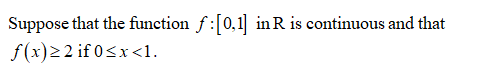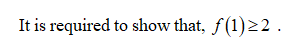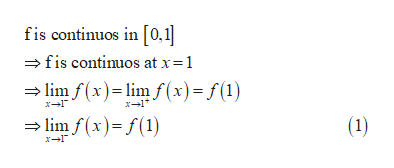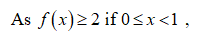# Suppose that the function f: [0, 1]  in R is continuous and thatf (x)>= 2     if 0 <= x < 1.Show that f(1) >= 2.

Question
2 views

Suppose that the function f: [0, 1]  in R is continuous and that
f (x)>= 2     if 0 <= x < 1.
Show that f(1) >= 2.

check_circle

Step 1Step 2help_outlineImage Transcriptionclosefis continuos in [0,1 =fis continuos at x=1 = lim f (x)= lim f(x)= f (1) = lim f(x)= f(1) X-1* (1) fullscreen
Step 3...

### Want to see the full answer?

See Solution

#### Want to see this answer and more?

Solutions are written by subject experts who are available 24/7. Questions are typically answered within 1 hour.*

See Solution
*Response times may vary by subject and question.
Tagged in

### Math